Next: 2.3.2 Nonlinear Effects in dx-y-Wave Up: 2.3 The Magnetic Field Previous: 2.3 The Magnetic Field

## 2.3.1 Nonlinear Effects in an s-Wave Superconductor

Since the high-Tc compounds are extreme type-II superconductors, they should be well described by the London theory. The underlying assumption in the linear relation between the supercurrent density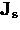and the vector potentialthat appears in the London model is that the applied field is both weak and slowly varying over a distance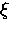. This in turn implies that the wave functions of the superconducting carriers are independent of magnetic field H and thatscales exactly with the velocity of the superfluid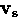[see Eq. (2.7)].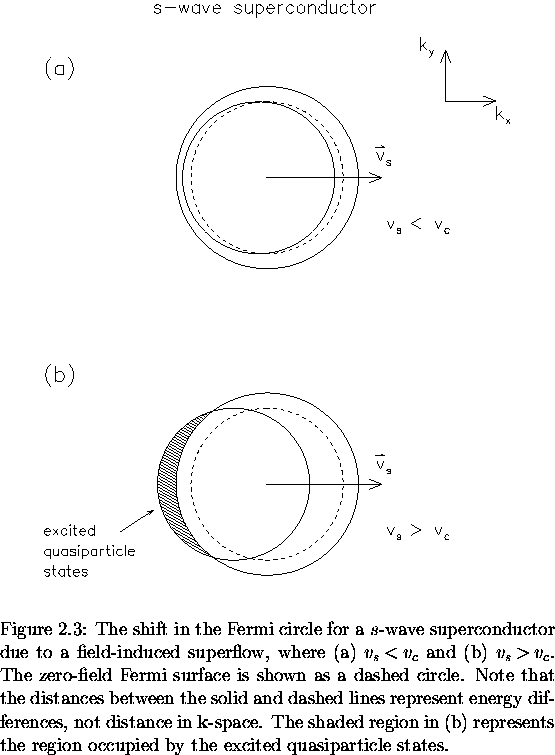Let us first consider an s-wave superconductor in the Meissner state for the case where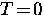, so that there is no contribution to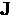from thermally excited quasiparticles [i.e. the second term in Eq. (2.22) is zero]. If a static magnetic field is then applied to the BCS ground state, each electron with initial momentum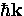will have a new momentum given by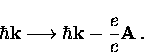(37)
The other electron in a pair has momentum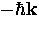, so that the new momentum of these electrons is simply(38)
The net effect is to shift the entire Fermi circle in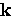-space as shown in Fig. 2.3.

The change in kinetic energy of an electron pair is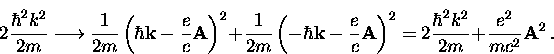(39)
Using Eq. (2.7) and Eq. (2.18) and summing up the contribution from all Cooper pairs gives the total increase in kinetic energy due to the applied magnetic field as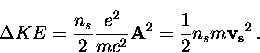(40)
This is just the classical equation for ns electrons per unit volume moving with a velocity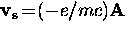. Thus, the supercurrents are determined solely by the applied field, and only exist in its presence. Provided the displacement of the Fermi circle does not exceed the width of the energy gap [as in Fig. 2.3(a)], the supercurrent response will remain linear. However, if the applied field is large enough, the shift will exceed this limit and quasiparticle excitations will occur. This latter case is illustrated in Fig. 2.3(b). Naturally, this will lead to a reduction in ns and a corresponding increase in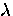. Near the Fermi surface, a quasiparticle has kinetic energy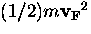. The change in kinetic energy of the quasiparticle due to the superflow velocityis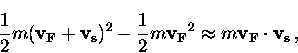(41)
so that there is a shift in the quasiparticle excitation spectrum such that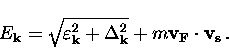(42)
The shift in quasiparticle energy relative to the energy gap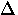,can be used to define a critical velocity for vs, below which the supercurrent response is linear in the Meissner state of an s-wave superconductor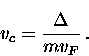(43)
Thus, ifthen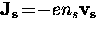. For velocities greater than vc, there is a quasiparticle contribution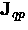such that the supercurrent density is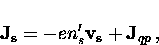(44)
where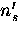is the reduced value of the superfluid density (relative to its maximum value ns in the linear regime). Thusdrop rapidly when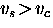so that the supercurrent response becomes nonlinear. If the energy shift is larger than the condensation energy (which will be derived later), the sample will become normal.

For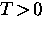, the size of the energy gap is reduced, so that the value of vc decreases. The precise form of the supercurrent-velocity relation of Eq. (2.45) is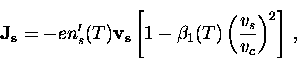(45)
where the prefactoris a T-dependent coefficient that decreases rapidly with T, with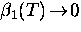as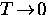.At low temperatures,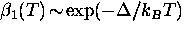.Using Eq. (2.46), the nonlinear London equations can be constructed to determine the field dependence of.It is found that for a conventional s-wave superconductor at low T,changes quadratically with H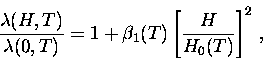(46)
where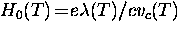is a characteristic field on the order of the thermodynamic critical field Hc(T), which is of course related to vc. Above H0 (T) the nonlinear effects become important. The H2 behaviour in Eq. (2.47) has been confirmed experimentally in the type-I superconductors Sn and In [31,32,33] and the type-II superconductor V3Si . The coefficientin Eq. (2.47) is typically small in conventional superconductors.Next: 2.3.2 Nonlinear Effects in dx-y-Wave Up: 2.3 The Magnetic Field Previous: 2.3 The Magnetic Field